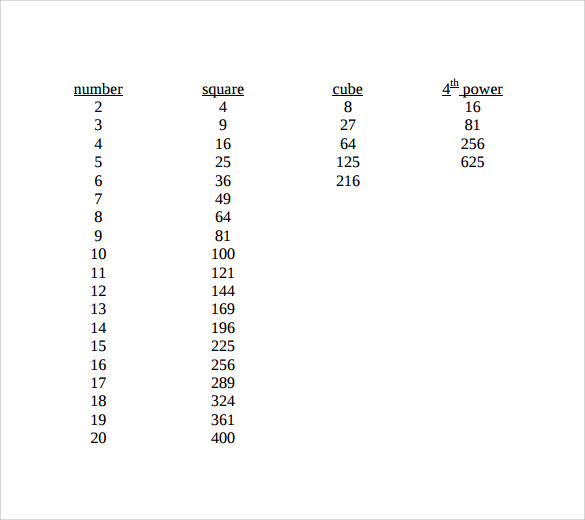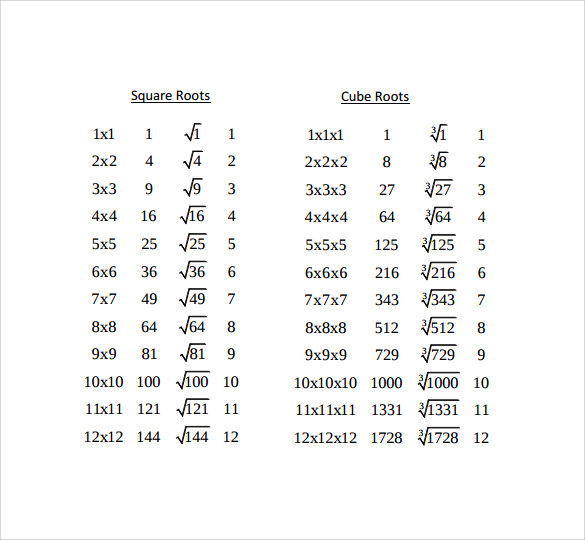Square root values have many mathematical applications. In higher mathematics too, square root is always significant. A square root chart normally contains square root and square values of 1-100. There are some square roots like that of 2, 3 or 7 which has no fixed value, but their square root values are shown in approximate decimal numbers.

From the chart the students can easily refer to these approximate square root values. The chart also teaches how to get a square root value of a number which is not shown in the chart. The chart helps the students to memorize some very common square root values to use them in other Math Worksheet Template problem solving processes.

## Sample Square Root Chart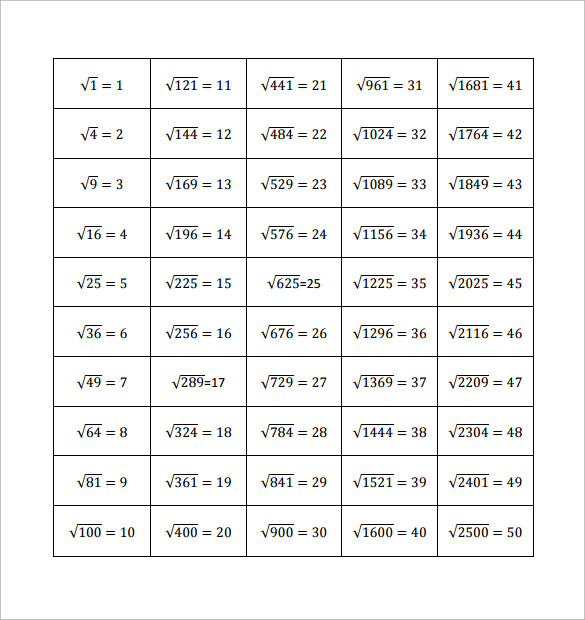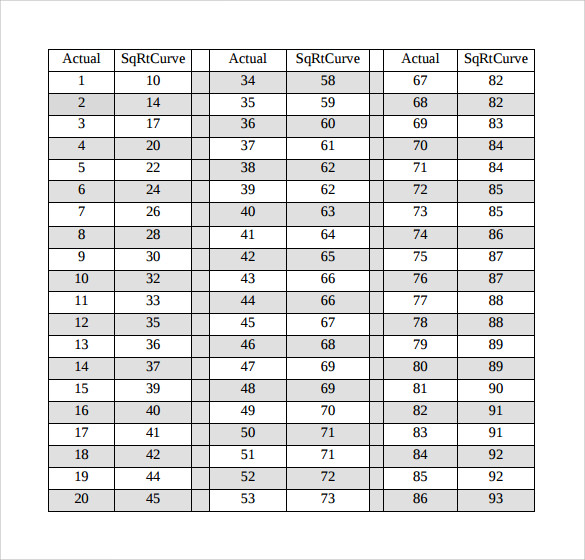## Simple Square Root Chart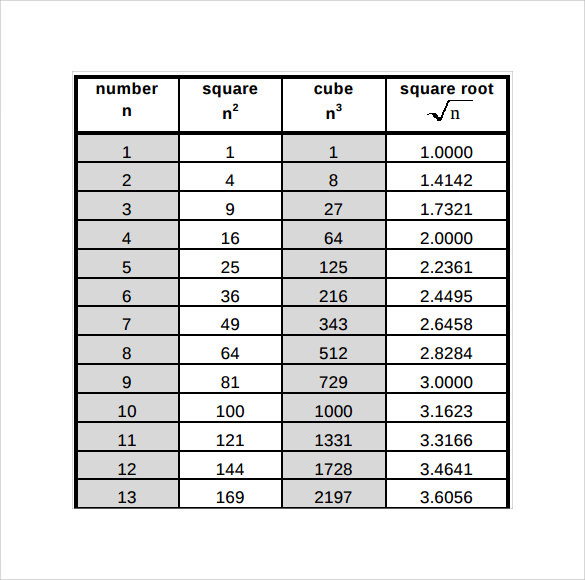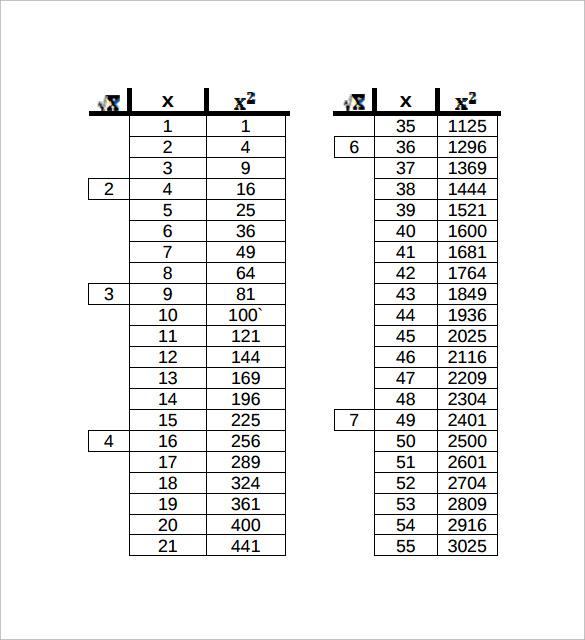## Square Root Chart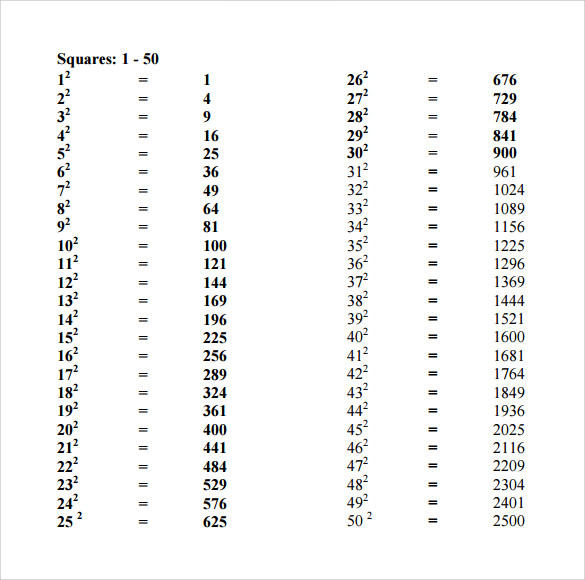## Example of Square Root Chart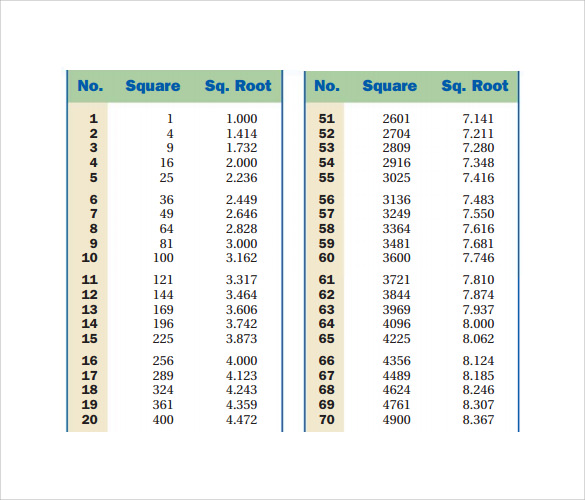## Square Root Chart In PDF